# Bihar Board Class 10th Maths Solutions Chapter 7 Coordinate Geometry Ex 7.4

Bihar Board Class 10th Maths Solutions Chapter 7 Coordinate Geometry Ex 7.4 Textbook Questions and Answers.

## BSEB Bihar Board Class 10th Maths Solutions Chapter 7 Coordinate Geometry Ex 7.4Question 1.
Determine the ratio in which the line 2x + y – 4 = 0 divides the line segment joining the points A(2, – 2) and B(3, 7).
Solution:
Suppose the line 2x + y – 4 = 0 divides the line segment joining A(2, – 2) and B(3, 7) in the ratio k : 1 at point C. Then, the coordinates of C are $$\left(\frac{3 k+2}{k+1}, \frac{7 k-2}{k+1}\right)$$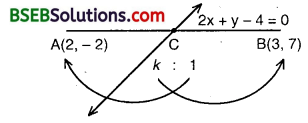But C lies on 2x + y – 4 = 0. Therefore,
2$$\left(\frac{3 k+2}{k+1}\right)$$ + $$\left(\frac{7 k-2}{k+1}\right)$$ – 4 = 0
or 6k + 4 + 7k – 2 – 4k – 4 = 0
or 9k – 2 = 0
or 9k = 2 i.e., $$\frac { 2 }{ 9 }$$
So, the required ratio is 2 : 9 internally.

Question 2.
Find a relation between x and y, if the points (x, y), (1, 2) and (7, 0) are collinear.
Solution:
The points A(x, y), B(1, 2) and C(7, 0) will be collinear, if
x(2 – 0) + 1(0 – y) + 7(y – 2) = 0
i.e., 2x – y + 7y – 14 = 0
or 2x + 6y – 14 = 0
or x + 3y – 7 = 0
which is the required relation between x and y.Question 3.
Find the centre of a circle passing through the points (6, – 6), (3, – 7) and (3, 3).
Solution:
Let P(x, y) be the centre of the circle passing through the points A(6, – 6), B(3, – 7) and C(3, 3).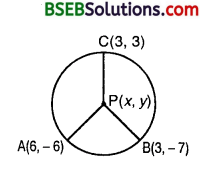Then, AP = BP = CP.
Now, AP = BP
So, AP² = BP²
So, (x – 6)² + (y + 6)² = (x – 3)² + (y + 7)²
or x² – 12x + 36 + y² + 12y + 36 = x² – 6x + 9 + y² + 14y + 49 or – 12x + 6x + 12y – 14y + 72 – 58 = 0
or – 6x – 2y + 14 = 0
or 3x +y – 7 = 0 … (1)
Also, BP = CP
So, BP² = CP²
So, (x – 3)² + (y + 7)² = (x – 3)² + (y – 3)²
or x² – 6x + 9 + y² + 14y + 49 = x² – 6x + 9 + y² – 6y + 9
or – 6x + 6x + 14y + 6y + 58 – 18 = 0
or 20y + 40 = 0
or y = $$\frac { -40 }{ 20 }$$ = – 2 …. (2)
Putting y = – 2 in (1), we get
3x – 2 – 7 = 0
or 3x = 9
or x = 3
Thus, the centre of the circle is (3, – 2).

Question 4.
The two opposite vertices of a square are (- 1, 2) and (3, 2). Find the coordinates of the other two vertices.
Solution:
Let ABCD be a square and let A(- 1, 2) and C(3, 2) be the given opposite vertices.
Let B(x, y) be the unknown vertex.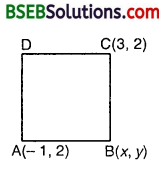Then, AB = BC gives AB² = BC²
So, (x + 1)² + (y – 2)² = (x – 3)² + (y – 2)²
or 2x + 1 = – 6x + 9
or 8x = 8
or x = 1 … (1)
In A ABC, we have : AB² + BC² = AC2. A(“
So, (x + 1)² + (y – 2)² + (x – 3)² + (y – 2)² = (3 + 1)² + (2 – 2)²
or 2x² + 2y² + 2x – 4y – 6x – 4y + 1 + 4 + 9 + 4 = 16
or 2x² + 2y² – 4x – 8y + 2 = 0
or x² + y² – 2x – 4y + 1 = 0 … (2)
Substituting the value of x from (1) in (2), we get
1 + y² – 2 – 4y + 1 = 0
or y² – 4y = 0
or y(y – 4) = 0
i. e., y – 0 or y = 4
Hence, the required vertices of the square are (1, 0) and (1, 4).Question 5.
The class X students of a secondary school in Krishinagar have been allotted a rectangular plot of land for their gardening activity. Saplings of Gulmohar are planted on the boundary at a distance of 1 m from each other. There is a triangular grassy lawn in the plot as shown in the figure. The students are to sow seeds of flowering plants on the remaining area of the plot.
(i) Taking A as origin, find the coordinates of the vertices of the triangle.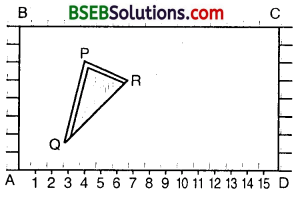(ii) What will be the coordinates of the vertices of ∆ PQR if C is the origin? Also, calculate the area of the triangle in these cases. What do you observe ?
Solution:
(i) Taking A as the origin, AD and AB are the coordinate axes. Clearly, the points P, Q and R are (4, 6), (3, 2) and (6, 5) respectively.
(ii) Taking C as the origin, CB and CD are the coordinate axes. Clearly, the points P, Q and R are given by (12, 2), (13, 6) and (10, 3) respectively.
We know that the area of the triangle whose vertices are (x1, y1), (x2, y2) and (x3, y3) is given by
$$\frac { 1 }{ 2 }$$[x1(y2 -y3) + x2(y3 -y1) + x3(y1 – y2)].
∴ Area of ∆ PQR in the 1st case
= $$\frac { 1 }{ 2 }$$[4(2 – 5) + 3(5 – 6) + 6(6 – 2)]
= $$\frac { 1 }{ 2 }$$(4 x (-3) + 3 x (- 1) + 6 x 4)
= $$\frac { 1 }{ 2 }$$(- 12 – 3 + 24) = $$\frac { 9 }{ 2 }$$sq. units
and Area of ∆ PQR in the 2nd case
= $$\frac { 1 }{ 2 }$$[12(6 – 3) + 13(3 – 2) + 10(2 – 6)]
= $$\frac { 1 }{ 2 }$$(12 x 3 + 13 x 1 + 10 x (-4))
= $$\frac { 1 }{ 2 }$$(36 + 13 – 40) = $$\frac { 9 }{ 2 }$$sq. units
Thus, we observe that the areas are the same in both the cases.

Question 6.
The vertices of a ∆ ABC are A(4, 6), B(l, 5) and C(7, 2). A line is drawn to intersect sides AB and AC at D and E respectively such that $$\frac { AD }{ AB }$$ = $$\frac { AE }{ AC }$$ = $$\frac { 1 }{ 4 }$$. Calculate the area of A ADE and compare it with the area of ∆ ABC.
Solution:
Using only Coordinate Geometry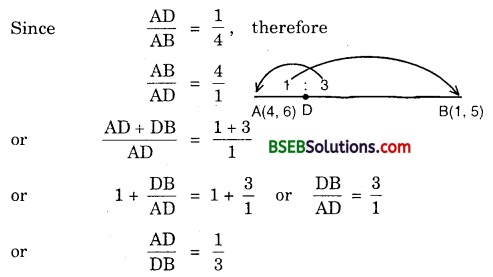Since D divides AB in the ratio 1:3, therefore coordinates of D are
$$\left(\frac{1 \times 1+3 \times 4}{1+3}, \frac{1 \times 5+3 \times 6}{4}\right)$$, i.e., ($$\frac { 13 }{ 4 }$$, $$\frac { 23 }{ 4 }$$)
From $$\frac { AE }{ AC }$$ = $$\frac { 1 }{4 }$$ , we find that
$$\frac { AE }{ AC }$$ = $$\frac { 1 }{ 3 }$$
Since E divides AC in the ratio 1:3, therefore coordinates of E are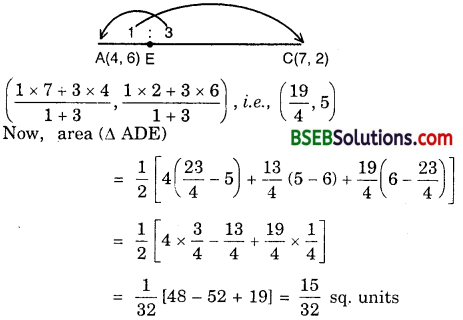Also, we find the area (∆ ABC) :
Area ∆ ABC = $$\frac { 15 }{ 2 }$$ sq. units [already found]
Area (∆ ADE) : Area (∆ ABC)
= $$\frac { 15 }{ 32 }$$ : $$\frac { 15 }{ 2 }$$ = $$\frac { 1 }{ 32 }$$ : $$\frac { 1 }{ 2 }$$ = 1 : 16

Aliter :
We know that if a line divides any two sides of a triangle in the same ratio, then the line is parallel to the third side.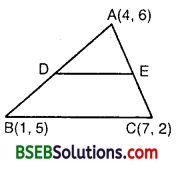∴ DE || BC
Clearly, A ADE ~ A ABC (Being equiangular, so similar)
We know that the ratio of the areas of two similar triangles is equal to the ratio of the squares of any two corresponding sides.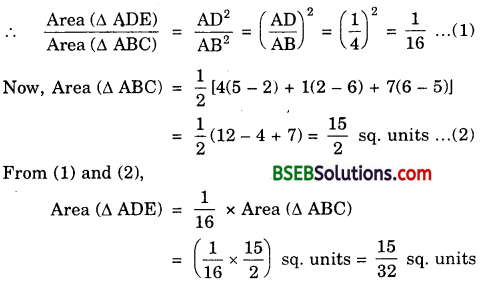Also, from (1), Area (∆ ADE) : Area (∆ ABC) = 1 : 16.Question 7.
Let A(4, 2), B(6, 5) and C(1, 4) be the vertices of ∆ ABC.
(i) The median from A meets BC at D. Find the coordinates of the point D.
(ii) Find the coordinates of the point P on AD such that AP : PD = 2:1.
(iii) Find the coordinates of points Q and R on medians BE and CF respectively such that BQ : QE = 2 : 1 and CR : RF = 2:1.
(iv) What do you observe?
(Note : The point which is common to all the three medians is called centroid and this point divides each median in the ratio 2 : 1.)
(v) If A(x1 y1), B(x2, y2) and C(x3, y3) are the vertices of ∆ ABC, find the coordinates of the centroid of the triangle.
Solution:
Let A(4, 2), B(6, 5) and C(1, 4) be the vertices of A ABC.
(i) Since AD is the median of ∆ ABC, therefore
D is the mid-point of BC. Its coordinates are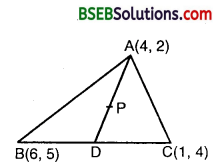$$\left(\frac{6+1}{2}, \frac{5+4}{2}\right)$$, i.e., $$\left(\frac{7}{2}, \frac{9}{2}\right)$$

(ii) Since P divides AD in the ratio 2 : 1, so its coordinates are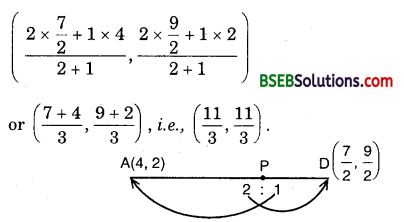(iii) Since BE is the median of ∆ ABC, so E is the mid-point of AC and its coordinates are E$$\left(\frac{4+1}{2}, \frac{2+4}{2}\right)$$, i.e., E($$\frac { 5 }{ 2 }$$, 3)Since Q divides BE in the ratio 2 : 1, so its coordinates areSince CF is the median of ∆ ABC, so F is the mid-point of AB. Therefore, its coordinates are F$$\left(\frac{4+6}{2}, \frac{2+5}{2}\right)$$. i.e., F(5, $$\frac { 7 }{ 2 }$$)
Since R divides CF in the ratio 2 : 1, so its coordinates are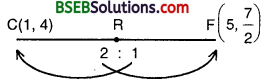Since R divides CF in the ratio 2 : 1, so its coordinates are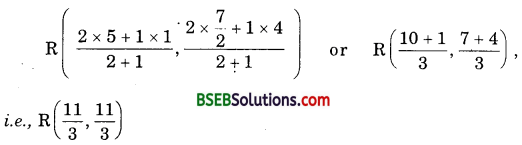(iv) We observe that the points P, Q and R coincide, i.e., the medians AD, BE and CF are concurrent at the point ($$\frac { 11 }{ 3 }$$, $$\frac { 11 }{ 3 }$$). This point is known as the centroid of the triangle.

(v) Let A(x1, y1, x2, y2) and C(x3, y3 be the vertices of ∆ ABC whose medians are AD, BE and CF respectively. So, D, E and F are respectively the mid-points of BC, CA and AB.The coordinates of E are $$\left(\frac{x_{1}+x_{3}}{2}, \frac{y_{1}+y_{3}}{2}\right)$$ coordinates of a point dividing BE in the ratio 2 : 1 are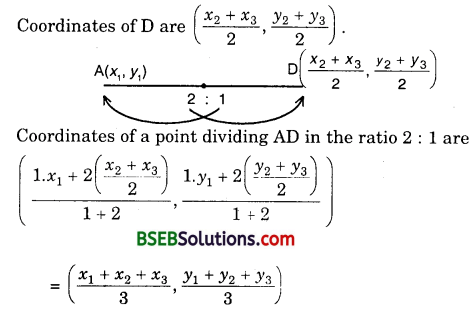The coordinates of E are $$\left(\frac{x_{1}+x_{3}}{2}, \frac{y_{1}+y_{3}}{2}\right)$$. The coordinates of a point dividing BE in the ratio 2 : 1 are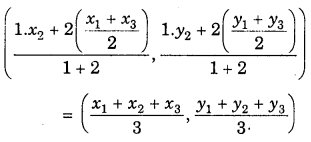Similarly, the coordinates of a point dividing CF in the ratio 2 : 1 are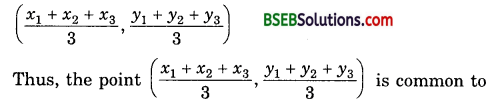AD, BE and CF and divides them in the ratio 2 : 1.
∴ The medians of a triangle are concurrent and the coordinates of the centroid are
$$\left(\frac{x_{1}+x_{2}+x_{3}}{3}, \frac{y_{1}+y_{2}+y_{3}}{3}\right)$$Question 8.
ABCD is a rectangle formed by joining the points A(- 1, – 1), B(- 1, 4), C(5, 4) and D(5, – 1). P, Q, R and S are the mid-points of AB, BC, CD and DA respectively. Is the quadrilateral PQRS a square? a rectangle? or a rhombus? Justify your answer.
Solution:
Various points are marked in the figure shown on the next page.Since all the sides are equal but the diagonals are not equal, therefore PQRS is a rhombus.